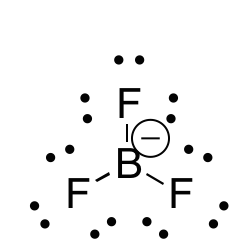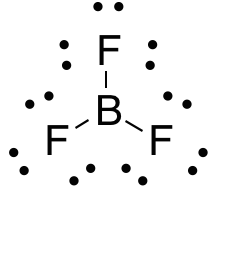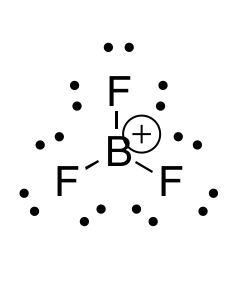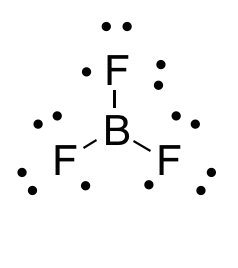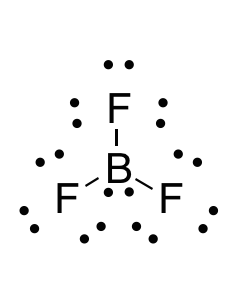# GRE Subject Test: Chemistry : Lewis Diagrams

## Example Questions

### Example Question #1 : Lewis Diagrams

Which answer option correctly depicts the Lewis dot structure of sodium chloride?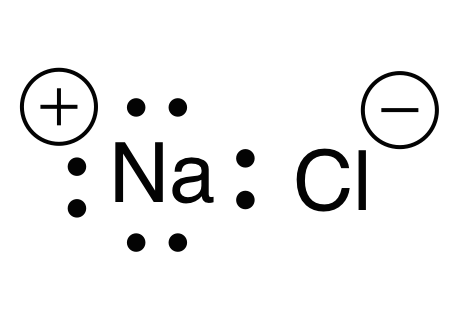Explanation:

When drawing a Lewis dot structure, we are always trying to reach an electron count where all atoms involved are stable and (usually) have full octets. We are also trying to estabilsh a structure in which we have the smallest formal charge possible. The general rule is first to draw out all of the elements involved and their valence electrons, then start piecing them together trying to reduce the formal charge and get all elements involved to an octet. There are a couple exceptions to the octet rule.

Sodium and chlorine form an ionic bond, meaning that one atom will donate an electron and the other will receive it. This gives each atom a charge. Chlorine has seven valence electrons, while sodium has one valence electron. For each atom to arrive at an octet, sodium will need to lose one electron and chlorine will need to gain one electron. This would give chlorine a negative charge, and sodium a positive charge.

Thus, the answer is a sodium with a positive charge (due to one lost electron) and a chlorine with eight electrons and a negative charge (due to one electron gained).

### Example Question #2 : Help With Lewis Diagrams

What is the Lewis dot structure for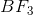?